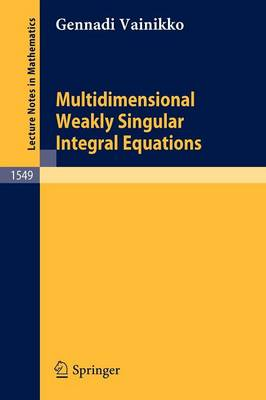•Multidimensional Weakly Singular Integral Equations - Lecture Notes in Mathematics 1549 (Paperback)

(author)
£22.99
Paperback 168 Pages / Published: 29/07/1993
• We can order this

Usually dispatched within 3 weeks

The final aim of the book is to construct effective discretization methods to solve multidimensional weakly singular integral equations of the second kind on a region of Rn e.g. equations arising in the radiation transfer theory. To this end, the smoothness of the solution is examined proposing sharp estimates of the growth of the derivatives of the solution near the boundary G. The superconvergence effect of collocation methods at the collocation points is established. This is a book for graduate students and researchers in the fields of analysis, integral equations, mathematical physics and numerical methods. No special knowledge beyond standard undergraduate courses is assumed.

Publisher: Springer-Verlag Berlin and Heidelberg GmbH & Co. KG
ISBN: 9783540568780
Number of pages: 168
Weight: 580 g
Dimensions: 235 x 155 x 9 mm
Edition: 1993 ed.